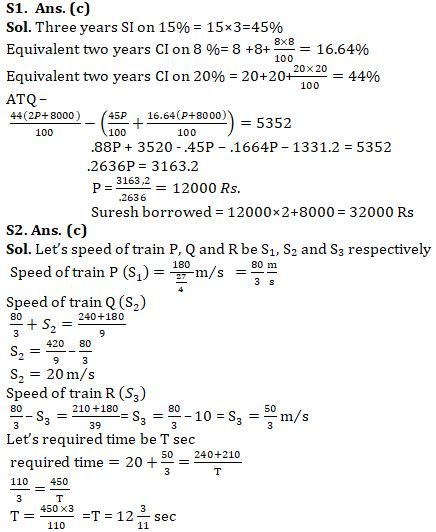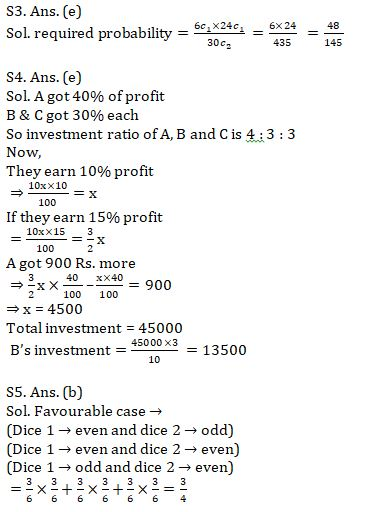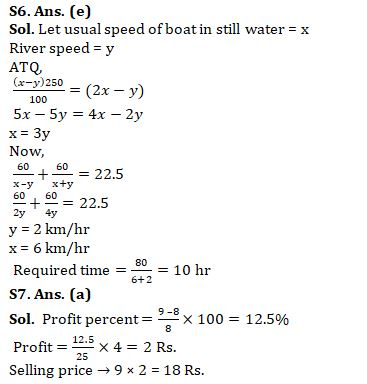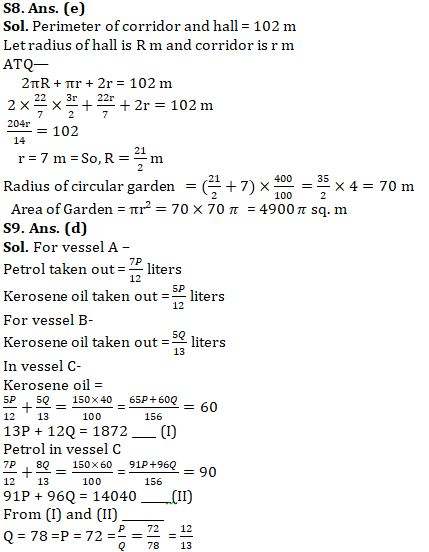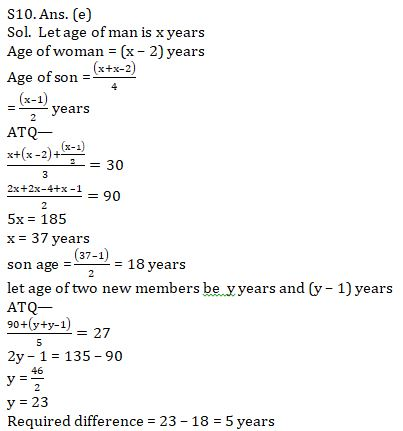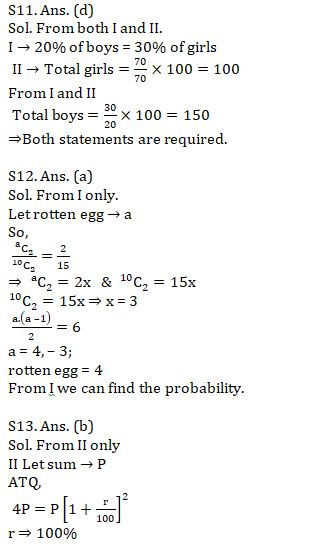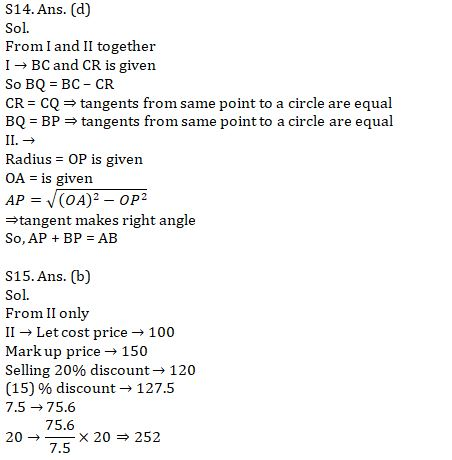Latest Banking jobs   »   Quantitative Aptitude Quiz For SBI Clerk...

# Quantitative Aptitude Quiz For SBI Clerk Mains 2021- 9th August

Q1. Manoj lend Rs. P for three years on S.I. at the rate of 15% per annum and Rajesh lend Rs. (P + 8000) for two years on C.I. at the rate of 8% per annum. Suresh borrowed sum equal to of what Manoj and Rajesh lend, for two years on C.I. at the rate of 20% per annum. If Suresh paid interest Rs. 5352 more than, what Manoj and Rajesh got total interest on their sums together. Find total sum borrowed by Suresh?
(a) Rs. 34000
(b) Rs. 44000
(c) Rs. 32000
(d) Rs. 46000
(e) Rs. 30000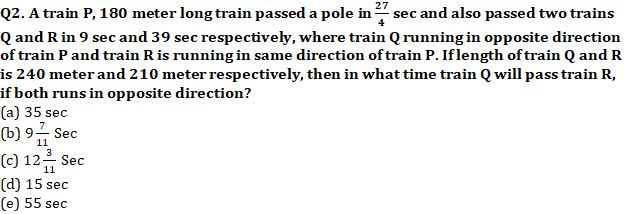Q3. A box contains 30 eggs out of which 6 are rotten. Two eggs are chosen at random. What is the probability that only one of the chosen eggs is rotten ?
(a) 53/145
(b) 63/145
(c)51/145
(d) 57/145
(e) 48/145

Q4. A, B and C entered into a partnership with some investment for one year. After one-year A got 2/5 profit and B and C got equal part of remaining profit. If total profit after one year is 15% instead of 10% then A got 900 Rs. more. Find the investment of B.
(a) 12000
(b) 45000
(c) 27000
(d) 18000
(e) 13500

Q5. Two dice are thrown, find the probability of getting an even number after multiplying numbers obtained from the dice.
(a) ¼
(b) ¾
(c) ⅔
(d) ½
(e) ⅙

Q6. A boat cover 60 km upstream and 60 km downstream in 22.5 hr with its usual speed. If boat double its speed then new upstream speed is 150% more than the usual upstream speed. Find the time taken by boat to cover 80 km in downstream with usual speed.
(a) 12 hr
(b) 20 hr
(c) 5 hr
(d) 16 hr
(e) 10 hr

Q7. Ratio between cost price and selling price of a article is 8 : 9 and ratio between numerical value of profit percent and numerical value of profit is 25 : 4. Find the selling price of the article.
(a) 18
(b) 27
(c) cannot be determined
(d) 16
(e) 24

Q8. In a house , there is a semicircular corridor, a circular hall and a circular garden. Sum of perimeter of corridor and hall is 102 m.If radius of hall is 50% more than radius of corridor then find area of circular garden, given radius of garden is 300% more than sum of radius of corridor and radius of hall together.
(a) 6300π sq.m
(b) 5400π sq.m
(c) 5700π sq.m
(d) 4200π sq.m
(e) 4900π sq.m

Q9. In vessel A mixture , petrol and kerosene oil are in the ratio of 7 : 5 and in vessel B it is in the ratio of 8 : 5. P liter of mixture from vessel A and Q liter of mixture from vessel B are taken out and poured into vessel C. If vessel C contains total 150 liter mixture with 40% kerosene oil, then find value of P/Q?
(a) 12/19
(b) 12/17
(c) 11/13
(d) 12/13
(e) 12/11

Q10. Average age of a man, woman and their son is 30 years. Man’s age is two year more than his wife and age of son is 1/4th the sum of age of his mother and father.When two other family members were added, new average becomes 27 years. If difference between age of two new member are one year then find difference between son and the new member who is elder.
(a) 7 years
(b) 8 years
(c) 4 years
(d) 2 years
(e) 5 years

Directions (11-15): The following questions are accompanied by two statements (i) and (ii). You have to determine which statement(s) is/are necessary/sufficient to answer the question.
Q11. In a group of boys and girls, 20% boys and 30% girls like the game chess. Find the total number of boys in the group.
(i) Number of boys like chess is equal to the number of girls like chess.
(ii) 70 girls present in the group do not like chess.
(a) Only (i)
(b) Only (ii)
(c) Only (i) or Only (ii).
(d) Both (i) and (ii) together required.
(e) Both (i) and (ii) are not sufficient

Q12. Find the probability of getting one rotten egg.
(i) Probability of getting 2 rotten egg from the total of 10 egg is 2/15.
(ii) Number of rotten egg is 2 less than the number of fresh egg.
(a) Only (i)
(b) Only (ii)
(c) Only (i) or Only (ii)
(d) Both (i) and (ii) together required.
(e) Both (i) and (ii) are not sufficient

Q13. Find the rate of interest.
(i) A sum invested on S.I. for 5 year becomes Rs5160.
(ii) A sum become 4 times after 2 year on C.I
(a) Only (i)
(b) Only (ii)
(c) Only (i) or Only (ii)
(d) Both (i) and (ii) together required.
(e) Both (i) and (ii) are not sufficient

Q14. A quadrilateral ABCD is drawn to circumscribe a circle having center O. Find the length of AB
(i) length of BC and CR is given
(ii) Radius of circle and length of OA is given.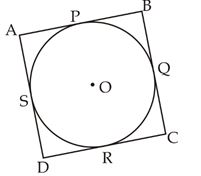(a) Only (i)
(b) Only (ii)
(c) Only (i) or Only (ii)
(d) Both (i) and (ii) together required.
(e) Both (i) and (ii) are not sufficient

Q15. Find the profit on selling an article?
(i) It is found that profit calculated on selling price is 20%.
(ii) Article is marked 50% above cost price and a discount of 20% is given on it. If 5% less discount is given, then he could have earn Rs.75.6 more.
(a) Only (i)
(b) Only (ii)
(c) Only (i) or Only (ii)
(d) Both (i) and (ii) together required.
(e) Both (i) and (ii) are not sufficient

Solution